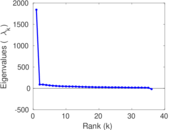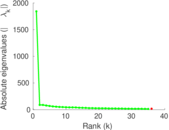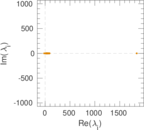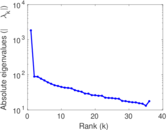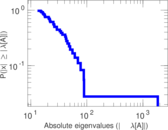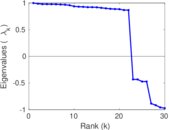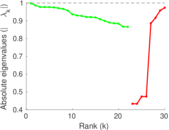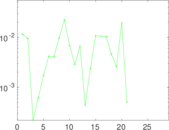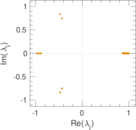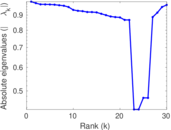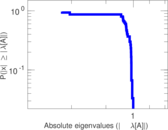This network consists of the wikilinks of the Wikipedia in the Sanskrit language (sa). Nodes are Wikipedia articles, and directed edges are wikilinks, i.e., hyperlinks within one wiki. In the wiki source, these are indicated with [[double brackets]]. Only pages in the article namespace are included.

 Code `Wsa` Internal name `wikipedia_link_sa` Name Wikipedia links (sa) Data source http://dumps.wikimedia.org/ AvailabilityDataset is available for download Consistency checkDataset passed all tests Category Hyperlink network Node meaning Article Edge meaning Wikilink Network format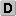Unipartite, directed Edge typeUnweighted, no multiple edges ReciprocalContains reciprocal edges Directed cyclesContains directed cycles Loops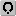Contains loops

## Statistics

 Size n = 21,779 Volume m = 3,720,076 Loop count l = 25 Wedge count s = 3,245,621,633 Claw count z = 15,483,354,545,477 Cross count x = 14,274,876,258,681,846 Triangle count t = 1,055,896,011 Square count q = 1,464,181,697,860 4-Tour count T4 = 11,726,440,048,038 Maximum degree dmax = 5,123 Maximum outdegree d+max = 1,855 Maximum indegree d−max = 4,878 Average degree d = 341.620 Size of LCC N = 21,628 Size of LSCC Ns = 12,588 Relative size of LSCC Nrs = 0.577 988 Diameter δ = 12 50-Percentile effective diameter δ0.5 = 3.280 32 90-Percentile effective diameter δ0.9 = 4.840 76 Median distance δM = 4 Mean distance δm = 3.840 85 Gini coefficient G = 0.895 358 Balanced inequality ratio P = 0.082 814 0 Outdegree balanced inequality ratio P+ = 0.074 618 4 Indegree balanced inequality ratio P− = 0.097 218 2 Power law exponent γ = 1.490 30 Degree assortativity ρ = +0.891 202 Degree assortativity p-value pρ = 0.000 00 In/outdegree correlation ρ± = +0.803 326 Clustering coefficient c = 0.975 988 Directed clustering coefficient c± = 0.999 132 Spectral norm α = 3,687.48 Operator 2-norm ν = 1,845.48 Algebraic connectivity a = 0.033 594 3 Spectral separation |λ1[A] / λ2[A]| = 9.810 76 Reciprocity y = 0.930 492 Non-bipartivity bA = 0.899 878 Normalized non-bipartivity bN = 0.012 375 9 Algebraic non-bipartivity χ = 0.074 466 0 Spectral bipartite frustration bK = 0.000 101 204

## Plots

### Fruchterman–Reingold graph drawing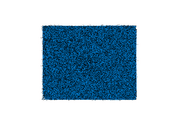### Degree distribution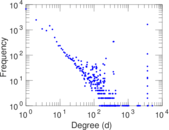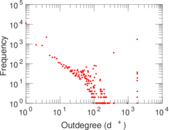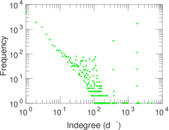### Cumulative degree distribution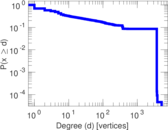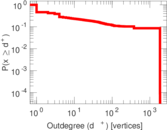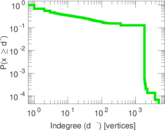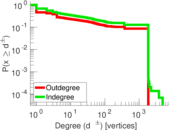### Lorenz curve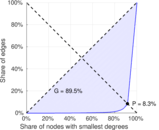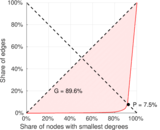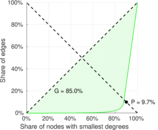### Spectral distribution of the adjacency matrix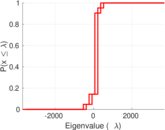### Spectral graph drawing based on the normalized adjacency matrix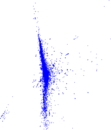### Degree assortativity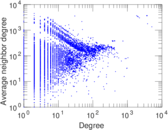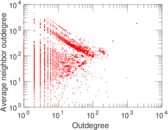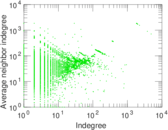### Zipf plot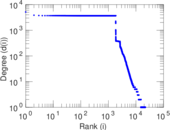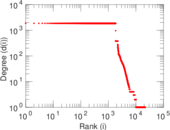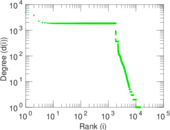### Hop distribution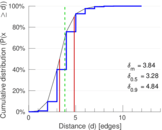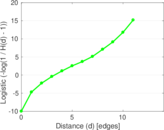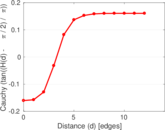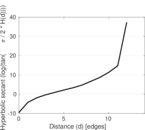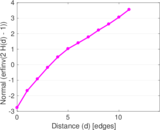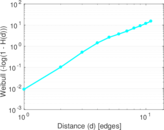### Delaunay graph drawing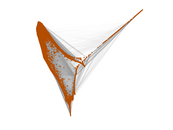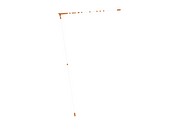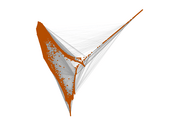### In/outdegree scatter plot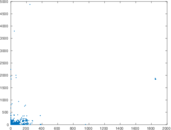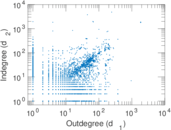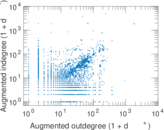### SynGraphy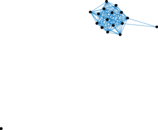### Matrix decompositions plots